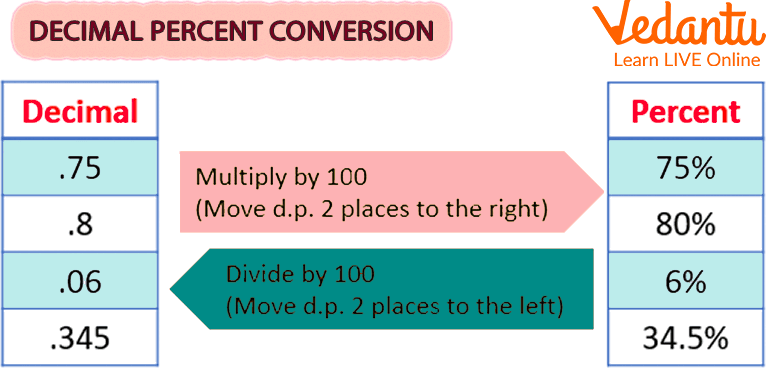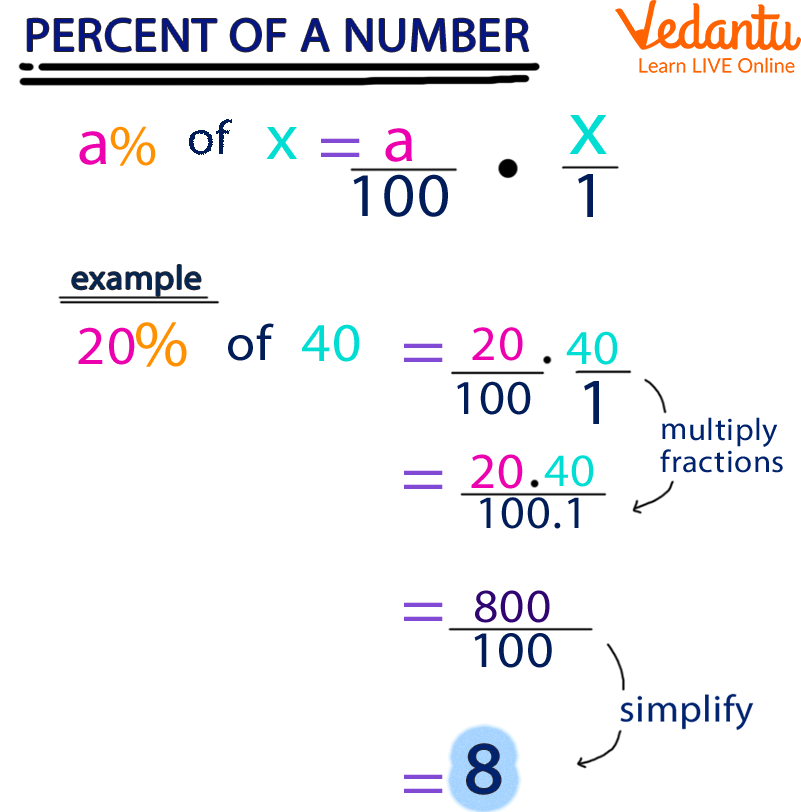Courses
Courses for Kids
Free study material
Free LIVE classes
More

# Conversion of Decimal to PercentageLIVE
Join Vedantu’s FREE Mastercalss

## Introduction to Decimals

A decimal number is one in which the whole number component and the fractional component are separated by a decimal point. The decimal point is the dot found in decimal numbers. The decimal point is followed by digits representing a number less than one.

Examples 1: 57.5 is a decimal number.

Here, 57 is a whole number part and 5 is the fractional part.

“.” is the decimal point.

Example 2: 78.9 is a decimal number

Here, 78 is a whole number part and 9 is the fractional part.

“.” is the decimal point.

In this article, it is explained how to convert decimal into percentages and how to convert a percentage to a number.

## Types of Decimal Numbers

Decimal Numbers can be classified as:

Recurring Decimal Numbers

Example: 3.125125 (Finite), 3.121212... (Infinite)

Non-Recurring Decimal Numbers

Example: 3.2376 (Finite), 3.137654 (Infinite)

Decimal Fraction

Example: $85.15=\frac{8515}{100}$

$32.5425 =\frac{325425}{10000}$

## How to Convert Decimal into Percentage?

The method for converting a decimal into a percentage is as follows:

Step 1: Get the number's decimal format.

Step 2: In this step, multiply the decimal amount by 100 and add the percent sign (%)

Remember: The decimal point must move two places to the right when multiplying a decimal number by 100. (add zeros if necessary).Decimal Percentage Conversion

## Percentage

A figure or ratio stated as a fraction of 100 is called a percentage. The percent sign, " %", is frequently used to indicate it. A percentage is a number without dimensions and without a standard measurement.

Example percentages include:

• 20 % is similar to a $\dfrac{1}{5}$ fraction

• 25% is equal to $\dfrac{1}{4}$ of a fraction

• 50% is equivalent to a $\dfrac{1}{2}$ fraction

• 75% is equal to a $\dfrac{3}{4}$ fraction

## Percentage Formula

To determine the percentage, we divide the data by the total value and then multiply the obtained value by 100.

Percentage Formula = $\dfrac{\text{Value}}{\text{Total Value}}\times100$

Example: $\dfrac{2}{5}\times 100% = 0.4 \times 100%=40 %$Convert Percentage to a Number

## How to Convert Percentage to Number?

To calculate the percentage of a number, we use the formula:

P % of Number = X, where X is the required percentage.

If we remove the symbol %, then the new formula will become;

$\dfrac{P}{100}\times Number=X$

From the above formula, it can be seen how to convert a percentage into a number.

## Solved Examples

Example 1: Calculate 20% of 80.

Ans: We will calculate the value by the given formula,

$\dfrac{P}{100}\times Number=X$

Let 20% of 80 = X

$\dfrac{20}{100}\times 80=X$

$X=16$

Example 2: Calculate 15% of 200.

Ans: We will calculate the value by the given formula,

$\dfrac{P}{100}\times Number=X$

Let 15% of 200 = X

$\dfrac{15}{100}\times 200=X$

$X=30$

## Practice Problems

Q1. Which of the following percentage is equivalent to 0.235?

1. 23.5%

2. 235.0%

3. 2.35%

4. None of the above

Q2. Express the given decimals as percentages-

1. 0.45

2. 0.067

3. 0.306

## Summary

In the given article, the conversion of decimal and percentage is discussed. This article gives us the basic knowledge of decimal, types of decimal, and related practice problems. Later, percentage and conversion tricks are given.

Last updated date: 27th Sep 2023
Total views: 124.5k
Views today: 1.24k

## FAQs on Conversion of Decimal to Percentage

1. Define percentage. Give examples.

A figure or ratio expressed as a fraction of 100 is called a percentage. The percent sign, "%", is used to indicate it.

Example percentages include:

• 20% is similar to a $\dfrac{1}{5}$ fraction

• 25% is equal to $\dfrac{1}{4}$ of a fraction

2. How to convert decimals into percentages? Convert 30.1 into a percentage.

The method for converting a decimal into a percentage is as follows.

Step 1. Get the number's decimal format.

Step 2.  In this step, multiply the decimal amount by 100 and add the percent sign (%)

The decimal number given in the question is 30.1.

In the next step, multiply the decimal amount by 100 and add the percent sign (%)

Therefore, $30.1\times 100% = 3010%$